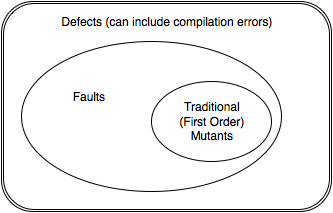According to IEEE 1044-2009 Standard Classification for Software Anomalies, A defect is a deficiency in the software artifact (the source code for us working in mutation analysis) that does not meet the requirements. A defect can be detected before execution of the code in question. If a defect escapes detection using any of the pre-execution techniques such as code-review, compilation, static analysis etc. it is called a fault. An error is a human action that can result in the above. That is, if a programmer understands a requirement incorrectly when producing the program, or the programming results in an oversight resulting in a deficiency. A fault is a manifestation of an error (and a single error can lead to many faults). A failure may be produced when a fault is encountered during execution of the code in question, and it is the deviation of the behavior of a system from its specification. A problem is an unsatisfactory interaction of a user with a system caused by a failure of the system to perform its function.

Mutation analysis requires an additional stage between faults and failures where the fault has been executed, and there is a difference in the state of the program from expected, but it has not yet resulted in a detectable behavioral change. To account for this, we typically call the human error a mistake, and the condition we just described a software error or more commonly, an error.

Mutants are faults that are injected. That is, we discount all defects that can be detected before execution of the program, and that includes defects that causes compilation errors or any defects detected by other automated means that does not involve actually running the code. This is an important distinction. Essentially mutants have to obey the grammar of the language in which the program is written in. If not, the number of trivially detectable mutants expands, providing very little value to the mutation score thus obtained. Obviously, the situation is a little fuzzy in the case of interpreted languages, where the interpreter may not detect the incorrect token until it is executed. However, it is recommended to closely follow this rule even in these languages.However, our neat classification system fails when it comes to equivalent mutants. These are mutants that do not necessarily encode a deficiency in the program, or if it does, the deficiency is masked by its use (i.e the fault will never become a failure, irrespective of inputs).

For example of the first variety, consider this.

def looper(j):
i = 0
while i < 10:
i++
j += i
return j

print looper(10)


An equivalent mutant is given by

def looper(j):
i = 0
while i < 10:
i++
j += i
return j

print looper(10)


The second version is identical to the first version. That is, the mutant is not a fault or even a defect. However consider this.

def roots(a,b,c):
d = b**2-4*a*c
if a == 0: return -a/b
if d < 0: return []
elif d == 0:
x1 = -b / (2*a)
return [x1]
else:
x1 = (-b + math.sqrt(d)) / (2*a)
x2 = (-b - math.sqrt(d)) / (2*a)
return [x1,x2]
roots(1,2,3)


It can be mutated to

def roots(a,b,c):
d = b**2-4*a*c
if d < 0: return []
elif d == 0:
x1 = -b / (2*a)
return [x1]
else:
x1 = (-b + math.sqrt(d)) / (2*a)
x2 = (-b - math.sqrt(d)) / (2*a)
return [x1,x2]
roots(1,2,3)


Since we only invoke root(1,2,3) the divide by zero can never be caused. However, intuitively, there is a mistake here, with programmer forgetting to check for the linear case. However, both this, and the previous mutant are under the equivalent mutant category.